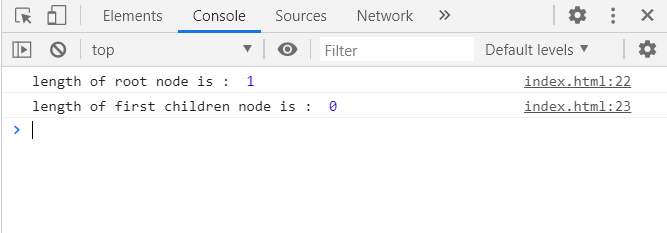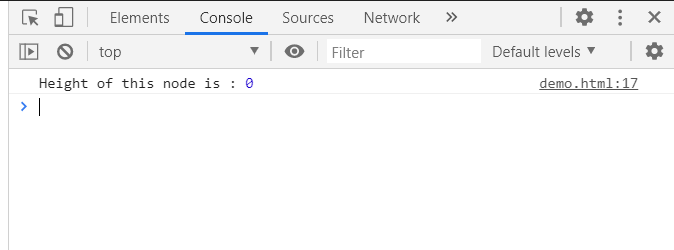Related Articles

# D3.js node.height Property

• Last Updated : 23 Sep, 2020

The node.height property in D3.js returns the greatest distance from the given node to a descendant leaf node.

Syntax:

`node.height`

Return Value: This property returns the greatest distance from the given node to a descendant leaf node

Example 1: In this example, for the root node to the leaf node the greatest distance is 1, for the leaf node the greatest distance is 0.

## HTML

 ```<``html``>`` ` `<``head``>``    ``<``meta` `charset``=``"utf-8"``>`` ` `    ``<``script` `src``=``        ``"https://d3js.org/d3.v5.min.js"``>``    `````` ` `<``body``>``    ``<``script``>``        ``var data = {``            ``"name":"GeeksforGeeks", ``            ``"about":"Computer Science Portal",``            ``"children":[``                ``{"name":"GFG1"},``                ``{"name":"GFG2"},``                ``{"name":"GFG3"},``                ``{"name":"GFG4"}``            ``]``        ``}``        ``var root = d3.hierarchy(data);``        ``console.log("Height of root node is : ",``                   ``root.height);``        ``console.log("Height of first children node is : ",``                   ``root.children.height);``    `````` ` ``

Output:Example 2: In this example, the hierarchy is not having any child so height is 0.

## HTML

 ```<``html``>``<``head``>``    ``<``meta` `charset``=``"utf-8"``>`` ` `    ``<``script` `src``=``        ``"https://d3js.org/d3.v5.min.js"``>``    `````` ` `<``body``>``    ``<``script``>``        ``var data = {"name":"GFG1"}`` ` `        ``var root = d3.hierarchy(data);`` ` `        ``console.log("Height of this node is :",``                    ``root.height);``    `````` ` ``

Output:My Personal Notes arrow_drop_up